# NORMDIST Function

Get the normal distribution for a stated mean and standard distribution

## What is the NORMDIST Function Excel Normal Distribution?

The NORMDIST function is categorized under Excel Statistical functions. It will return the normal distribution for a stated mean and standard distribution. That is, it will calculate the normal probability density function or the cumulative normal distribution function for a given set of parameters.

To understand what a normal distribution is, consider an example. Suppose we take an average of 30 minutes to commute to the office daily, with a standard deviation of 5 minutes. Assuming a normal distribution for the time it takes to go to work, we can calculate the percentage of time that the commuting time would be between 25 minutes and 35 minutes.

As a financial analyst, the NORMDIST function is useful in stock market analysis. When investing, we need to balance risk and return and aim for the highest possible return. Normal distribution helps quantify the amount of return and risk by the mean for return and standard deviation for risk.

### Formula

=NORMDIST(x,mean,standard_dev,cumulative)

The NORMDIST function uses the following arguments:

1. X (required argument) – This is the value for which we wish to calculate the distribution.
2. Mean (required argument) – The arithmetic mean of the distribution.
3. Standard_dev (required argument) – This is the standard deviation of the distribution.
4. Cumulative (required argument) – This is a logical value. It specifies the type of distribution to be used: TRUE (Cumulative Normal Distribution Function) or FALSE (Normal Probability Density Function).

The formula used for calculating the normal distribution is: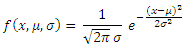Where:

• μ is the mean of the distribution
• σis the variance, and x is the independent variable for which you want to evaluate the function
• The Cumulative Normal Distribution function is given by the integral, from -∞ to x, of the Normal Probability Density function.

### How to use the NORMDIST Function in Excel?

To understand the uses of the NORMDIST function, let’s look at an example:

#### Example – Normal Distribution Excel

Suppose we are given the following data:

• Value for which we need distribution: 52
• Arithmetic mean of the distribution: 50
• Standard deviation of the distribution: 2.5

If we wish to calculate the cumulative distribution function for the data above,  the formula to use is: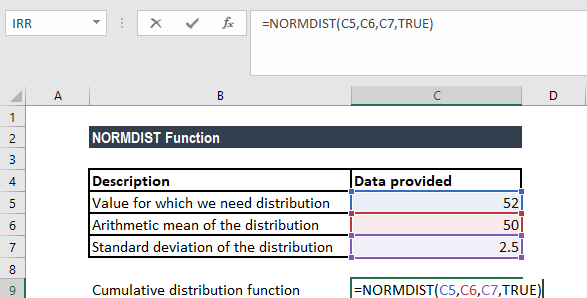We get the result below: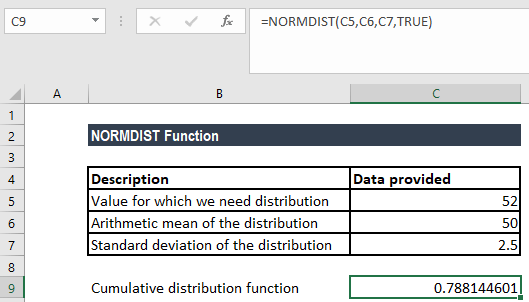If we wish to calculate the probability mass function for the data above, the formula to use is: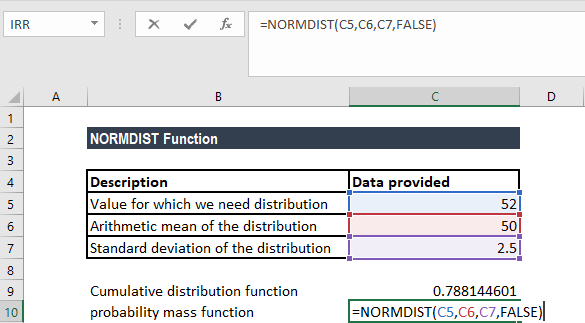We get the result below: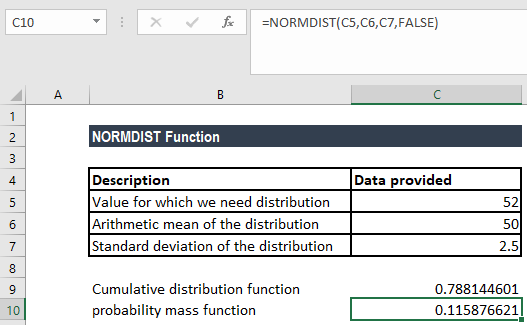### Notes about NORMDIST Normal Distribution in Excel

1. The NORMDIST function was replaced by NORM.DIST function in Excel 2010. NORM.DIST provides more accuracy than the NORMDIST function. The NORMDIST function is still available in Excel 2010 version. However, it is stored in the list of compatibility functions to allow compatibility with earlier versions of Excel.
2. The NORM.DIST function refers to any normal distribution, whereas the NORMSDIST compatibility function and the NORM.S.DIST function refer specifically to the unit normal distribution.
3. #NUM! error – Occurs if the given standard_dev argument is less than or equal to zero.
4. #VALUE! error – Occurs when any of the given arguments is non-numeric or is a non-logical value.
5. If mean = 0, standard_dev = 1, and cumulative = TRUE, NORMDIST returns the standard normal distribution, NORMSDIST.

Thanks for reading CFI’s guide to the Excel NORMDIST function. By taking the time to learn and master these functions, you’ll significantly speed up your financial analysis. To learn more, check out these additional CFI resources:

• Excel Functions for Finance